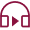Last Updated: 2022-06-15T21:19:54+05:30## Introduction

Before learning about futures price calculation, it is wise the know about futures contracts. A futures contract is a standardised legal agreement between a seller and a buyer. A futures contract makes it binding for the seller to sell and the buyer to buy an underlying asset at a specific date in the future. All futures trading strategies move around the difference in the futures price vis-a-vis the underlying asset's price. Understanding the futures pricing formula is crucial to know the contract's fair value before buying or selling.

## Futures Contract Formula Explained

The price of all futures contracts depends on the underlying asset. For example, the futures price of NIFTY is determined by the value of the NIFTY index. If the underlying asset price increases, the futures contract will most likely increase and vice versa. But, the price of the futures contract is generally not the same as the price of the underlying asset. The difference between a futures contract price and the underlying asset's price is known as the 'Spot Future Parity.'

So, why is the price of a futures contract typically different from the underlying asset price and what is the futures price calculation formula?

The answer to the question lies hidden in the date of contract expiration, interest rate, dividend, and the time to expiry. So, the price of a futures contract of the same underlying asset price is different across expiry dates. Let's understand this with an example.

Suppose, on 1st July, you buy three NIFTY futures contracts of 18000CE for three different expiry dates - 30th July, 30th August, and 30th September, when the spot price of NIFTY is 17000. Although the strike price (18000) remains the same across all three expiry dates, the price of the 30th September contract will be higher than 30th August. Similarly, the price of the 30th August contract will be higher than 30th July. This is because of the time value of the contract price to the expiry date.

Factors like the ones stated above affect the futures pricing formula. Therefore, the futures contract formula refers to the mathematical representation of a futures contract price in relation to the market dynamics or variables.

Here is the futures pricing formula for calculating the price of a futures contract:

Futures Price = Spot price *(1+ rf – d)

Here, 'rf' means the risk-free rate and 'd' means dividend. rf is what you might earn without any risk through a year. For example, if you decide to buy the futures contract of an asset whose spot price is 1000, the risk-free rate is 8%, and the days to expiry is 7 days. So, the futures price will be as per the following futures pricing formula:

Futures Price = 1000 * [1+ 8*(7/365) – d]

Using this futures contract formula, you can find the fair value of any futures contract of any expiry before applying your trading strategies. If you still find a difference between the fair value and the market price, blame it on transaction charges, brokerage fees, margin charges, and the like.

## Experience Super-Profitable Futures Trading With 5paisa

5paisa is a preferred gateway for people willing to trade in the futures market. Give your futures pricing formula wings with ultra-low brokerage fee and free Demat and trading account. Remember to pick some futures trading strategies before trading, though.

Resend OTP